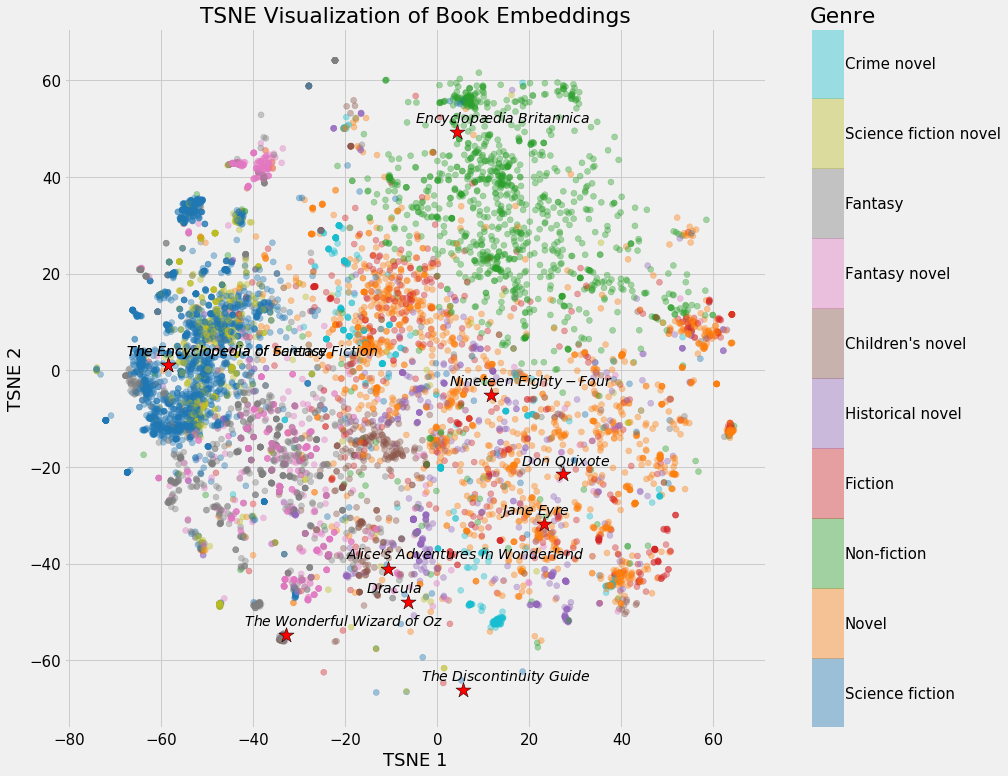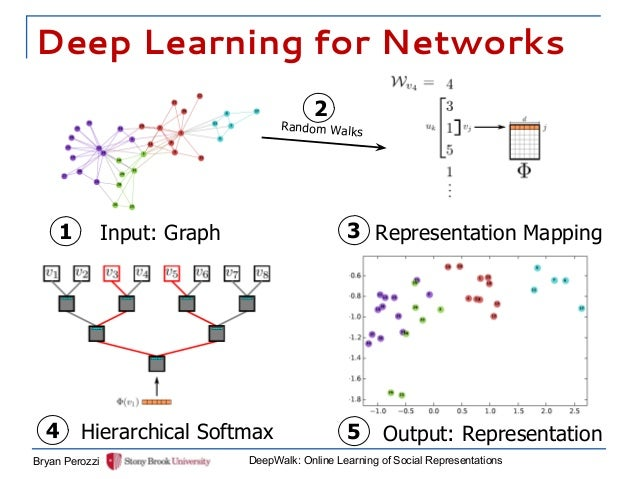# Low Dimensional Embedding

Low dimensional embedding is a method which maps the vertices of a graph into a low dimension vector space under certain constraint.These approaches typically first construct the affinity graph using the feature vectors of the data points, e.g., the K-nearest neighbor graph of data, and then embed the affinity graph into a low dimensional space. However, these algorithms usually rely on solving the leading eigenvectors of the affinity matrices, the complexity of which is at least quadratic to the number of nodes, making them inefficient to handle large-scale networks.

The first-order proximity in a network is the local pairwise proximity between two vertices. For each pair of vertices linked by an edge (u, v), the weight on that edge, wuv, indicates the firstorder proximity between u and v. If no edge is observed between u and v, their first-order proximity is 0. The first-order proximity usually implies the similarity of two nodes in a real-world network.

 The secondorder proximity between a pair of vertices (u, v) in a network is the similarity between their neighborhood network structures. Mathematically, let pu = (wu,1, . . . , wu, V ) denote the first-order proximity of u with all the other vertices, then the second-order proximity between u and v is determined by the similarity between pu and pv. If no vertex is linked from/to both u and v, the second-order proximity between u and v is 0

# Multidimensional scaling (MDS)

MDS is used to translate “information about the pairwise ‘distances’ among a set of n objects or individuals” into a configuration of n points mapped into an abstract Cartesian space.

Steps of a Classical MDS algorithm:

• Set up the squared proximity matrix $D$
• Apply double centering $B=-\frac{1}{2}JDJ$ with $J=I-\frac{1}{n}11^T$
• Determine the largest k eigenvalues and corresponding eigenvectors of B, where k is the number of dimensions desired for the output).
• The final feature is $X=E\Lambda^{\frac{1}{2}}$

# isomap

Isomap is one representative of isometric mapping methods, and extends metric multidimensional scaling (MDS) by incorporating the geodesic distances imposed by a weighted graph, instead of purely relies on pairwise distance. A very high-level description of Isomap algorithm is given below.

• Determine the neighbors of each point.
• All points in some fixed radius.
• K nearest neighbors.
• Construct a neighborhood graph.
• Each point is connected to other if it is a K nearest neighbor.
• Edge length equal to Euclidean distance.
• Compute shortest path between two nodes.
• Dijkstra’s algorithm
• Floyd–Warshall algorithm
• Compute lower-dimensional embedding.
• Multidimensional scaling

# Laplacian Eigenmap

Laplacian Eigenmaps uses spectral techniques to perform dimensionality reduction. This technique relies on the basic assumption that the data lies in a low-dimensional manifold in a high-dimensional space. This algorithm cannot embed out-of-sample points.

Assume $W$ is the adjacency matrix, Laplacian Eigenmaps formulates the embedded problem as a generalized eigen problem:

$Lf=\lambda Df$

where $D$ is a diagnoal matrix with $D_ii=\sum_j{W_{j,i}}$, $L=D-W$ and $f$ will be the feature of a dimension.

# Locally Linear Embedding (LLE)

• Find neighbours in X space
• Solve for reconstruction weights W
• for i=1:N
• create matrix Z consisting of all neighbours of Xi [d]
• subtract Xi from every column of Z
• compute the local covariance C=Z’*Z [e]
• solve linear system C*w = 1 for w [f]
• set Wij=0 if j is not a neighbor of i
• set the remaining elements in the ith row of W equal to w/sum(w);
• end
• Compute embedding coordinates Y using weights W
• create sparse matrix M = (I-W)’*(I-W)
• find bottom d+1 eigenvectors of M (corresponding to the d+1 smallest eigenvalues)
• set the qth ROW of Y to be the q+1 smallest eigenvector (discard the bottom eigenvector [1,1,1,1…] with eigenvalue zero)

# DeepWalk

DeepWalk is a novel approach for learning latent representations of vertices in a network. These latent representations encode social relations in a continuous vector space, which is easily exploited by statistical models.

DeepWalk uses local information obtained from truncated random walks to learn latent representations by treating walks as the equivalent of sentences.

• generate $\gamma$ random walks for each vertex
• random walk has length t
• the next step is picked randomly from its neighbors
• each walk is represented by a vector of the indices of the nodes in the walk
• find the feature representation maximalizes the likelihood of all the walksWritten on April 22, 2019